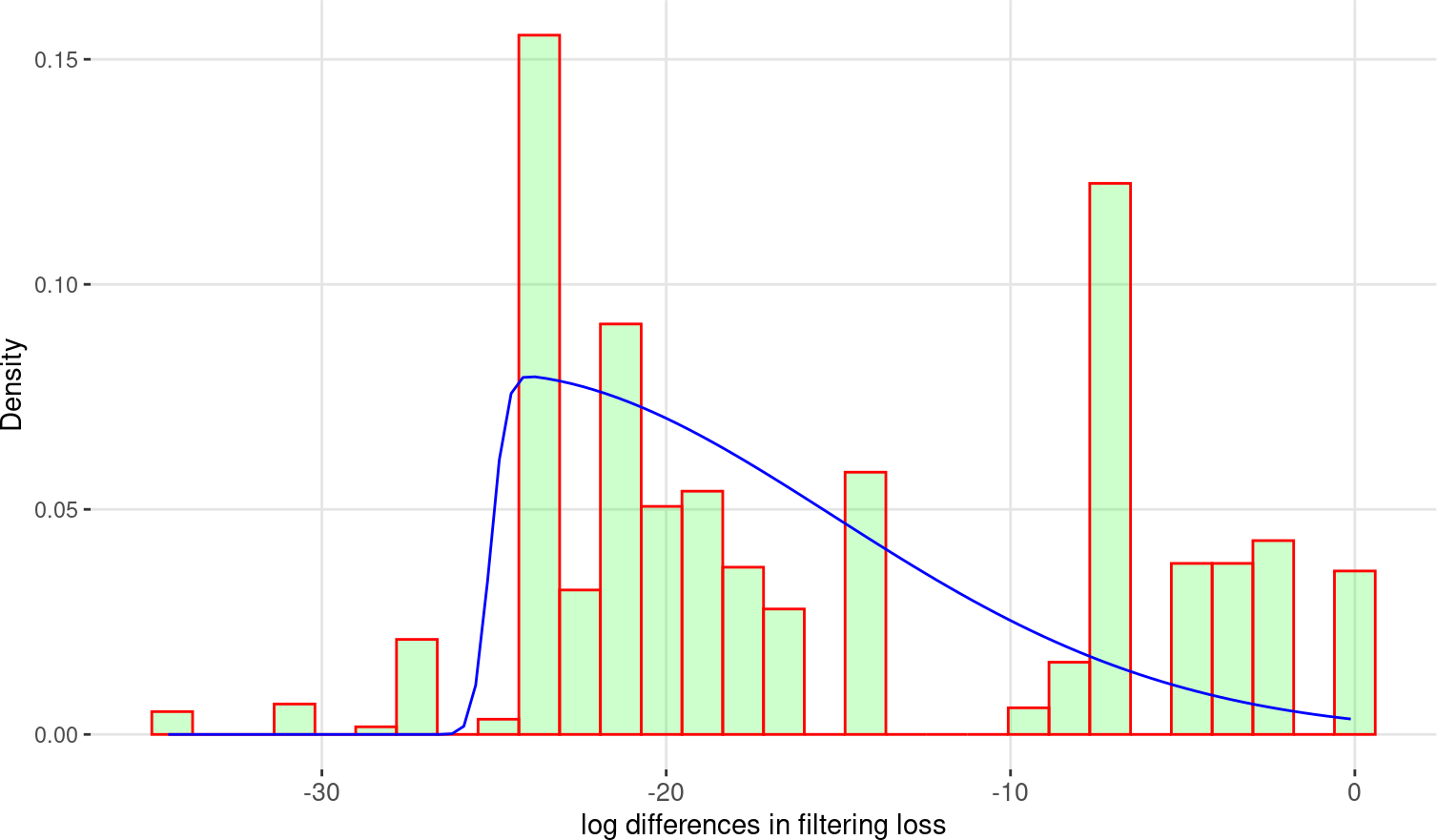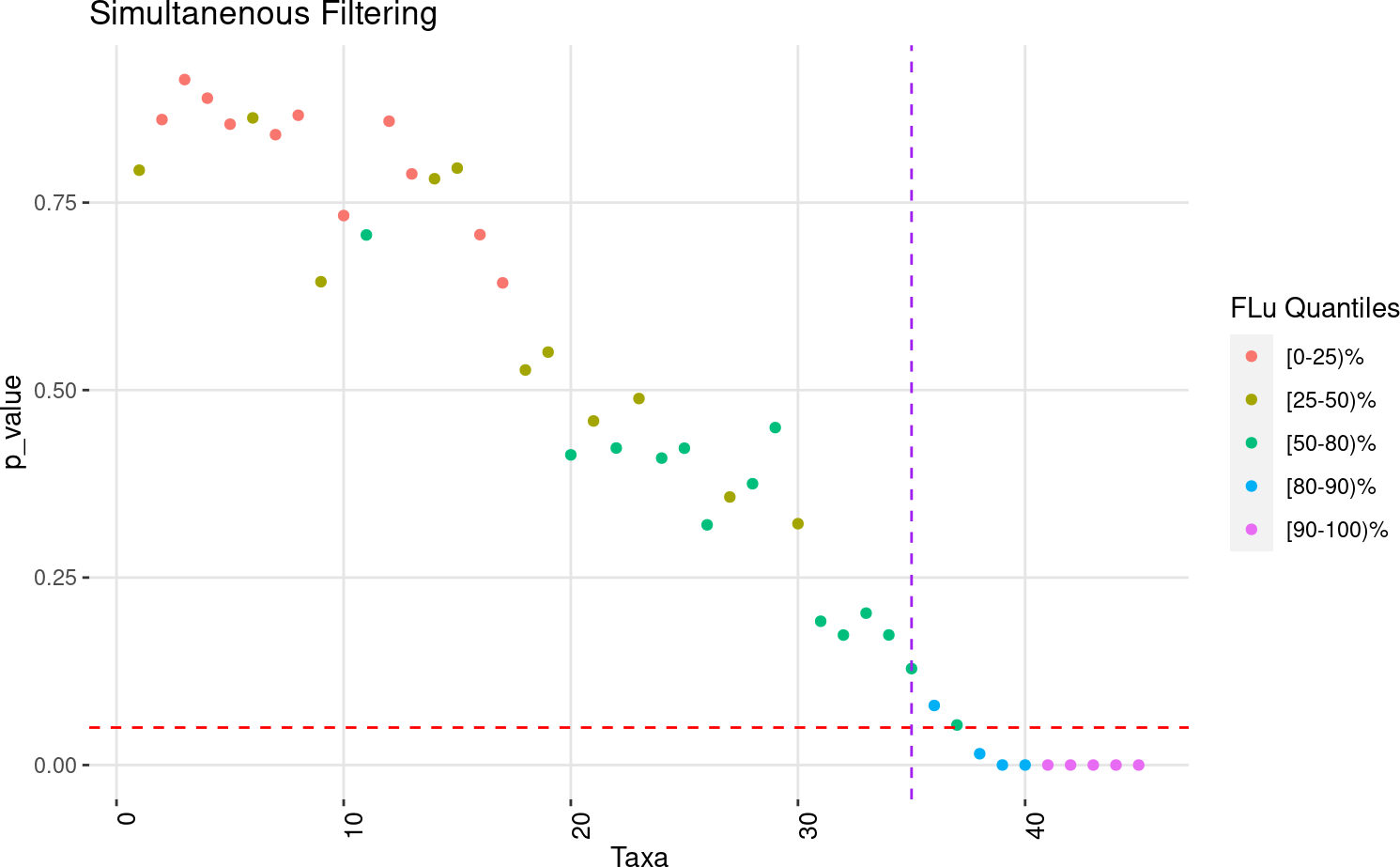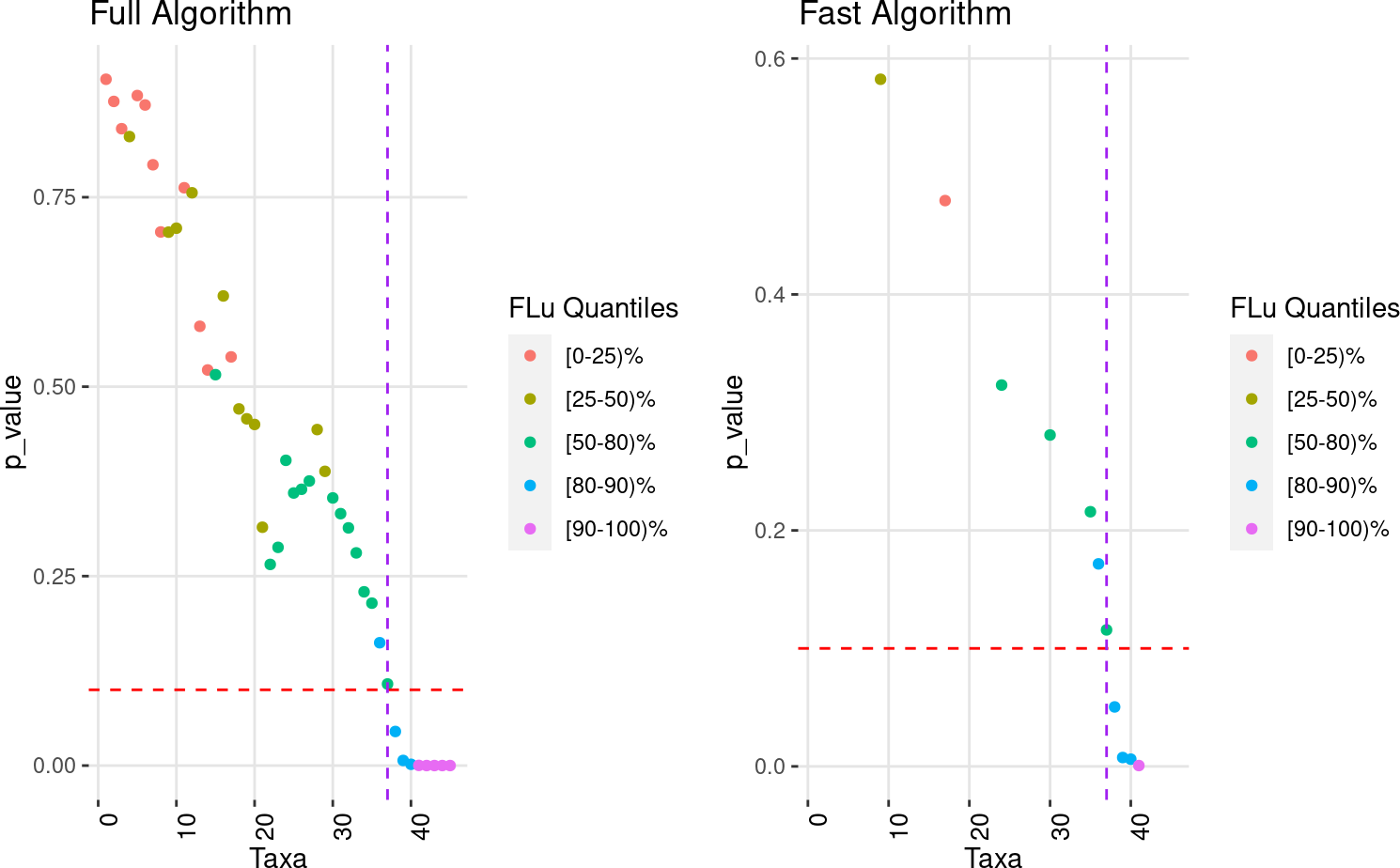# 1 Installation

The PERFect software is freely available at Bioconductor and can be installed using the following codes:

if(!requireNamespace("BiocManager", quietly = TRUE))
install.packages("BiocManager")
BiocManager::install("PERFect")

The following libraries are needed to reproduce the same results:

rm(list=ls())

library(PERFect)
library(ggplot2)
library(knitr)
library(kableExtra)
set.seed(12341)

# 2 Introduction

Next generation sequencing (NGS) of the 16S rRNA marker is currently among the most widely used methods for microbial organisms identification. In these studies, samples collected at different body sites (e.g., vaginal swab, stool or blood) give counts of DNA fragments which are then grouped into similar microbial organisms, usually referred to as taxa. Recent microbiome quality control studies show that majority of rare taxa are caused by contamination and/or sequencing errors. The most common approach to address this problemis filtering, or removing spurious taxa from the 16S data set. This vignette explains the idea of filtering loss $$FL(J)$$ and demonstrates the PERFect permutation method on a small subset of the mock community data (Brooks et al. 2015). There are in total $$10$$ samples ($$n = 10$$) and $$20$$ taxa ($$p = 20$$) which contain $$7$$ signal taxa. The two tables below shows ordered taxa abundance by columns, where the first table gives $$13$$ noise taxa and the second table gives $$7$$ signal taxa. For example, $$N_1$$ is the least abundant noise taxon and $$N_{13}$$ is the most abundant noise taxon; $$S_1$$ is the least abundant signal taxon and $$S_7$$ is the most abundant signal taxon.

N1 N2 N3 N4 N5 N6 N7 N8 N9 N10 N11 N12 N13
Sample 1 0 0 0 0 6 0 0 0 0 1 0 0 0
Sample 2 0 0 0 0 1 0 0 0 0 0 0 0 1
Sample 3 0 0 0 0 0 0 1 0 0 0 0 3 1
Sample 4 0 0 0 0 0 0 0 0 0 0 0 0 2
Sample 5 0 9 0 0 0 2 0 0 3 24 3 36 4
Sample 6 0 0 0 0 0 0 0 0 0 0 1 0 1
Sample 7 0 0 0 0 0 1 0 0 0 4 3 1 0
Sample 8 1 0 1 1 0 0 0 1 0 0 0 0 0
Sample 9 0 0 0 0 0 0 0 0 1 0 1 1 1
Sample 10 0 0 0 0 0 0 2 4 0 0 0 0 0
S1 S2 S3 S4 S5 S6 S7
Sample 1 2422 0 2 4971 5493 0 1
Sample 2 0 1 12725 663 0 4926 3
Sample 3 3307 0 0 3252 2 3 2
Sample 4 0 3199 0 0 0 1854 6501
Sample 5 1 19 4 51332 2 1 14
Sample 6 0 0 14212 0 883 7 11
Sample 7 1020 1 1 0 2 0 23306
Sample 8 2094 45 12 1 4 47 14188
Sample 9 0 16037 0 1557 2217 5 30
Sample 10 14 17 4559 3770 2 25 4836

# 3 Method Illustration

## 3.1 Filtering loss function

The filtering loss measures taxa contribution to the total covariance. We assume that if the set of $$J$$ taxa is not important, then removing these taxa will not dramatically decrease the OTU table total covariance (defined in section 2.1 of Smirnova et al. 2018). We define the loss due to filtering out the set of $$J$$ taxa as $FL (J_j)= 1- \frac{\text{covariance magnitude after removing J taxa}}{\text{total covariance}}.$

The filtering loss $$FL(J_j)$$ is a number between $$0$$ and $$1$$, with values close to $$0$$ if the set of taxa $$J$$ has small contribution to the total covariance and $$1$$ otherwise.

To find filtering loss, taxa are first re-arranged in ascending order from left to right according to their number of occurrences in the $$n$$ samples, i.e. the abundant taxa are to the right of the table. Once taxa are ordered, the filtering loss is calculated sequentially by removing the taxa from left to right.

We evaluate the $$j^{th}$$ taxon contribution to the signal using the difference in filtering loss statistic $DFL(j + 1) = FL(J_{j+1}) - FL(J_j).$ The values of $$DFL(j + 1)$$ are close $$0$$ if the $$j^{th}$$ taxon is included erroneously, and significantly different from $$0$$ if this taxon has high contribution to the signal.

To illustrate the ability of this filtering loss to distinguish between noise and signal taxa, the filtering loss values for a noise taxon, a signal taxon and a combination of noise and signal taxa are shown in the table below. Specifically, this table compares the filtering loss values and their corresponding log values due to removing:

1. The $$10^{th}$$ least abundant noise taxon, $$J_1 = \{N_{10}\}$$
2. The first least abundant signal taxon $$J_2 = \{S_1\}$$
3. All noise taxa, $$J_3 = \{N_1, \dots, N_{13}\}$$
4. All noise taxa and the first least abundant signal taxon, $$J_4 = \{N_1, \dots, N_{13}, S_1\}$$.

From the table, one can notice a large difference between log filtering loss value of the $$10^{th}$$ least abundant noise taxon and that of the first least abundant signal taxon ($$-14.786$$ v.s. $$-6.970$$ respectively). This implies that the data loss due to removing $$N_{10}$$ is minimal and dramatically less than the loss due to removing $$S_1$$. Moreover, since the loss from omitting all noise taxa is still less the loss from omitting one signal taxon, it shows that all noise taxa cumulatively have less contribution than a single signal taxon.

Filtering Loss Log Filtering Loss
N10 3.7876e-07 -14.786
S1 9.3960e-04 -6.970
Noise taxa 1.3109e-06 -13.545
Noise taxa and S1 9.4091e-04 -6.969

## 3.2 Permutation Filtering algorithm

This algorithm’s objective is to remove noise taxa while identifying and retaining signal taxa. It takes an OTU table and a test critical value $$\alpha$$ as inputs and produces a reduced OTU table with less taxa. The following pseudocode example illustrates this algorithm.

Input: OTU table above, test critical value $$\alpha = 0.1$$.

Step 1. Run simultaneous PERFect algorithm to obtain taxa p-values $$p_j, j=1, \dots, p$$.

The simultaneous PERFect algorithm yields preliminary taxa significance. Taxa can also be ordered by abundance and this step is not necessary; however method evaluation on mock data has shown that ordering taxa by simultaneous PERFect p-values improves permutation PERFect performance.

Step 2. Order columns of $$X$$ such that $$p_1 \geq p_2 \geq p_p$$.

The table below shows taxa and their corresponding simultaneous PERFect p-values. Some taxa that were initially less important according to the the abundance ordering gained higher rank according to simultaneous PERFect p-values. For example, the $$2^{nd}$$ least important taxon $$N_2$$ in the abundance ordering becomes the $$7^{th}$$ least important taxon in the simultaneous PERFect p-values ordering.

N1 N3 N4 N5 N6 N7 N2 N8 N9 N11 N10 N12 N13
Sim pvalues NA 0.87 0.8 0.8 0.8 0.79 0.77 0.61 0.55 0.52 0.45 0.34 0.24
S6 S4 S5 S1 S3 S2 S7
Sim pvalues 0.13 0.09 0.09 0.06 0.06 0.03 0.03

Step 3.

For taxon $$j = 1, \dots, p-1$$:

• Let $$J_j = \{1, \dots, j\}$$

• Calculate $$DFL(j+1) = FL(J+1) - FL(J)$$.

The test statistic $$DFL$$ and its corresponding values on the log scale for each taxon are displayed below. The values of $$\log(DFL)$$ range between $$-23.71$$ and $$-13.98$$ for the noise taxa and increase dramatically to $$-6.79$$ for the value of the first signal taxon $$S_6$$. The $$\log(DFL)$$ values for signal taxa range between $$-7.5$$ and $$-0.12$$, which is much larger compared to corresponding statistic values for the noise taxa.

N1 N3 N6 N4 N5 N7 N2 N8
DFL NA 5.06e-11 5.06e-11 5.94e-10 2.73e-09 7.49e-11 5.25e-08 4.16e-10
log(DFL) NA -23.71 -23.71 -21.24 -19.72 -23.31 -16.76 -21.6
N9 N11 N10 N12 N13
DFL 6.02e-09 7.28e-09 3.79e-07 8.51e-07 1.13e-08
log(DFL) -18.93 -18.74 -14.79 -13.98 -18.3
S6 S5 S4 S3 S1 S2 S7
DFL 1.12e-03 8.90e-01 5.52e-04 7.67e-04 1.83e-02 8.92e-03 8.08e-02
log(DFL) -6.79 -0.12 -7.5 -7.17 -4 -4.72 -2.52

For taxon $$j = 1, \dots, p-1$$:

• For permutation $$1, \dots, k$$:

+ Randomly select $$J^*_{j+1} \subset \{1, \dots, p\}$$ with $$|J^*_{j+1}| =j+1$$

+ Calculate $$DFL^*(j+1) = FL(J^*_{j+1}) - FL(J^*)$$.

In this step of the algorithm, we construct the permutation distribution for each set of $$j$$ taxa to evaluate significance of corresponding $$\log(DFL)$$ values. To build the distribution of $$d\mathcal{F}_{j+1}$$ and test statistical hypothesis $\begin{equation*} {\small H_0: d\mathcal{F}_{j+1} = 0 \hspace{10mm}\mbox{vs}\hspace{10mm} H_A: d\mathcal{F}_{j+1} > 0, } \end{equation*}$

we randomly select $$k$$ sets $$J^*_{j+1}$$ of taxa labels and calculate the corresponding $$DFL^*(j+1)$$ value for each sample. For example, to obtain 6 permutations ($$k = 6$$) for $$5$$ taxa, we draw sets of size $$|J^*_{j+1}| = 5$$. Then, the $$DFL^*$$ for these samples are calculated according to this permutation ordering. In particular, for the first permutation,

$DFL^*(5) = FL^*(\{N_8, N_{11}, S_2, S_6, N_4\}) - FL^*(\{N_8, N_{11}, S_2, S_6\}).$

The values of $$DFL^*$$ for other sets of taxa are calculated similarly.

Permutation 1 Permutation 2 Permutation 3 Permutation 4 Permutation 5 Permutation 6
Taxa 1 N1 N2 S4 N12 S4 N3
Taxa 2 S7 S2 N8 S3 N9 S2
Taxa 3 N3 N5 N9 S2 N8 N12
Taxa 4 N2 N10 N7 S6 N3 S1
Taxa 5 S1 S5 N4 N5 N7 N13
log(DFL*) -3.94 -0.12 -21.84 -19.72 -23.31 -18.33

For taxon $$j = 1, \dots, p-1$$:

• Using quantile matching, fit the normal distribution to the logarithm of the sample $$DFL^*(j+1), j = 1, \dots, p-1$$ to obtain the null distribution $$X_j \sim \mbox{SN}(\widehat\xi_j, \widehat\omega_j^2, \widehat\alpha_j)$$.

Here, after $$k$$ values of $$\log(DFL^*)$$ for each group of $$j$$ taxa are obtained, they are fit to a Skew Normal distribution with three parameters $$\xi,\omega$$ and $$\alpha$$ using quantile matching method. For example, to estimate the parameters of $$5$$ taxa distribution, we would use $$\log(DFL^*)$$ values from the $$6$$ columns in the table above to fit Skew Normal distribution to the sample $$\{-21.25,-23.71,-4.7,-13.98,-3.95,-6.79\}$$.

This step is necessary to disentangle the null and alternative distributions contributing to the observed permutation distribution. When we use $$k$$ permutations to build the reference distribution of filtering loss differences, it always includes both the values from the null and from the alternative hypotheses as illustrated above in the example of step 4 of the algorithm. Therefore, we need to make the assumption that the resulting permutation distribution is a mixture of observed differences under the null and alternative distributions. The p-value is a measure of how extreme a value of statistic (here $$DFL(5)$$) is relative to the null distribution. If we use fully non-parametric approach and simply construct the p-value as the proportion of times when $$\log DFL(5)$$ is above $$\log DFL^*(5)$$, then we use mixture of null and alternative as the null distribution. Under this approach, the p-value would not be appropriately calculated and we may lose more taxa than necessary.In this example, we increase the number of permutations to $$k=1000$$, which is necessary to get a reasonable distribution for the values of $$DFL^*(j+1)$$. The histogram of $$5$$ taxa distribution is given below with the blue line indicating Skew Normal fit with parameters ($$\hat{\xi}, \hat{\omega},\hat{\alpha}$$) = (-25.12, 9.95, 26.19). Since we have only $$20$$ taxa in this example, the distribution fit is not as accurate as for the larger number of taxa, but it illustrates the idea of the algorithm.

For taxon $$j = 1, \dots, p-1$$:

• Calculate the p-value $$p_j$$ for $$DFL(j+1), j = 1, \dots, p-1$$ as $$p_j := P[X_j > \log\{DFL(j+1)\}]$$.

The $$\log(DFL)$$ value for the $$5^{th}$$ taxon in simultaneous p-values ordering was calculated in step 3 as -19.72. Therefore, we calculate the $$5^{th}$$ taxon p-value as $$p_{5} = P[X_{5} > -19.72]$$ = 0.583, where $$X_{5} \sim \mbox{SN}(\widehat\xi_{5} = -25.26, \widehat\omega_{5}^2 = 10.09, \widehat\alpha_{5} = 34.41)$$.

1. Average $$3$$ subsequent p-values

The $$4$$ rows of the example OTU table below combines taxa PERFect simultaneous p-values, their corresponding $$\log(DFL)$$ values, raw PERFect permutation p-values, and their corresponding averaged values. The p-values of noise taxa are large and the p-values for the signal taxa are small as expected.

N1 N3 N4 N5 N6 N7 N2 N8 N9 N11 N10 N12 N13
Sim pvalues NA 0.87 0.80 0.80 0.80 0.79 0.77 0.61 0.55 0.52 0.45 0.34 0.24
log(DFL) NA -23.71 -23.71 -21.24 -19.72 -23.31 -16.76 -21.60 -18.93 -18.74 -14.79 -13.98 -18.30
Perm pvalues NA 0.90 0.90 0.69 0.59 0.82 0.39 0.65 0.51 0.43 0.18 0.09 0.45
Avg Perm pvalues NA 0.83 0.73 0.70 0.60 0.62 0.52 0.53 0.38 0.23 0.24 0.18 0.15
S6 S4 S5 S1 S3 S2 S7
Sim pvalues 0.13 0.09 0.09 0.06 0.06 0.03 0.03
log(DFL) -6.79 -0.12 -7.50 -7.17 -4.00 -4.72 -2.52
Perm pvalues 0.00 0.00 0.05 0.06 0.04 0.01 0.00
Avg Perm pvalues 0.02 0.04 0.05 0.04 0.02 0.01 0.00
1. Filter the set of taxa $$J_j$$ with the first p-value such that $$p_{j+1} \leq \alpha$$

The OTU table above indicates that the first significantly small averaged p-value is $$p_{14}= 0.02 \leq 0.1 :=\alpha$$, which occurs at the first signal taxon $$S_6$$. Thus the algorithm has successfully filtered noise taxa and preserved all the true signal taxa.

# 4 Workflow

The filtering methods for this package are wrapped into two main functions, PERFect_sim() (performing simultaneous filtering) and PERFect_perm() (performing permutation filtering). First, we load the OTU matrix with 240 samples and 46 taxa.

data(mock2)
Counts <- mock2$Counts dim(Counts) ##  240 46 By default, the function PERFect_sim() takes the data table, $$X$$, as a matrix or data frame, orders it by the taxa abundance, uses 10%, 25% and 50% quantiles for matching the log of DFL to a Skew-Normal distribution and then calculates the p-value for each taxon at the significance level of $$\alpha$$ = 0.1. The function PERFect_sim() only needs a taxa table as the input, and other parameters are set to default. res_sim <- PERFect_sim(X = Counts) Sometimes, the input taxa table may contain metadata for each samples, such as the location where each sample is processed, the total reads for each sample or simply patient data. Such information must be pointed out in the input table, using the argument “infocol”. For example, if the metadata were located at the first 5 columns, the syntax would be: res_sim <- PERFect_sim(X = Counts, infocol = c(1,2,3,4,5)) Although there are four provided taxa ordering, user can order taxa the way they wanted using the argument “Order.user”. Specifically, user will provide a character vector of ordered taxa names. For example, if we wanted to order taxa by alphabet (which is the original ordering of the taxa table), we would input the following codes: alphabet.ordering <- colnames(Counts) head(alphabet.ordering) ##  "Aerococcus.christensenii" ##  "Agromyces.cluster54" ##  "Atopobium.vaginae" ##  "Bacteroides.massiliensis" ##  "Bifidobacterium.longum_infantis_suis" ##  "Bosea.cluster53" res_sim <- PERFect_sim(X = Counts, Order.user = alphabet.ordering) The “filtX” object from the result stores the filtered OTU matrix, which consists of 240 samples and 10 taxa in this example. We can identify the remaining taxa by looking into the column of the OTU matrix. dim(res_sim$filtX)      
##  240  10
colnames(res_sim$filtX) # signal taxa ##  "Methylophilus.cluster11" "Mobiluncus.mulieris" ##  "Pseudomonas.gessardii" "Gardnerella.vaginalis" ##  "Prevotella.bivia" "Atopobium.vaginae" ##  "Lactobacillus.crispatus_cluster" "Streptococcus.agalactiae" ##  "Lactobacillus.iners" "Sneathia.amnii" The p-values for all taxa can be extracted as head(res_sim$pvals)
## Bifidobacterium.longum_infantis_suis              Coriobacteriaceae.OTU27
##                              0.79319                              0.86069
##                              0.91403                              0.88922
##               Lactobacillus.jensenii                Mycoplasma.agalactiae
##                              0.85453                              0.86292

and they can be plot using the function pvals_Plots().

p <- pvals_Plots(PERFect = res_sim, X = Counts, quantiles = c(0.25, 0.5, 0.8, 0.9), alpha=0.05)
p$plot + ggtitle("Simultanenous Filtering")Alternatively, we can use permutation filtering PERFect_perm() which is more robust than simultaneous filtering. By default, this function generates k = 10000 permutations for each taxa, thus it can be computationally expensive for a large OTU matrix. We offer user a fast algorithm which employs an unbalanced binary search that optimally finds the cutoff taxon without building the permutation distribution for all taxa. The codes for these options are shown below. res_perm <- PERFect_perm(X = Counts, Order = "pvals", pvals_sim = res_sim, algorithm = "full") res_perm2 <- PERFect_perm(X = Counts, Order = "pvals", pvals_sim = res_sim, algorithm = "fast") p1 <- pvals_Plots(res_perm, Counts) p1 <- p1$plot + ggtitle("Full Algorithm")
p2 <- pvals_Plots(res_perm2, Counts)
p2 <- p2\$plot + ggtitle("Fast Algorithm")
ggpubr::ggarrange(p1,p2,ncol = 2)The figure above illustrates the plot of permutation PERFect p-values calculated by the full and fast algorithm for the mock2 dataset. Although both methods achieve the similar cutoff taxon, the fast algorithm only calculate 11 out 46 p-values hence is more computationally efficient.

# 5 Session Info

## R version 4.1.1 (2021-08-10)
## Platform: x86_64-pc-linux-gnu (64-bit)
## Running under: Ubuntu 20.04.3 LTS
##
## Matrix products: default
## BLAS:   /home/biocbuild/bbs-3.14-bioc/R/lib/libRblas.so
## LAPACK: /home/biocbuild/bbs-3.14-bioc/R/lib/libRlapack.so
##
## locale:
##   LC_CTYPE=en_US.UTF-8       LC_NUMERIC=C
##   LC_TIME=en_GB              LC_COLLATE=C
##   LC_MONETARY=en_US.UTF-8    LC_MESSAGES=en_US.UTF-8
##   LC_PAPER=en_US.UTF-8       LC_NAME=C
##  LC_MEASUREMENT=en_US.UTF-8 LC_IDENTIFICATION=C
##
## attached base packages:
##  stats4    stats     graphics  grDevices utils     datasets  methods
##  base
##
## other attached packages:
##  kableExtra_1.3.4 knitr_1.36       ggplot2_3.3.5    PERFect_1.8.0
##  sn_2.0.0         BiocStyle_2.22.0
##
## loaded via a namespace (and not attached):
##    colorspace_2.0-2       ggsignif_0.6.3         ellipsis_0.3.2
##    rio_0.5.27             XVector_0.34.0         rstudioapi_0.13
##    ggpubr_0.4.0           farver_2.1.0           fansi_0.5.0
##   xml2_1.3.2             codetools_0.2-18       splines_4.1.1
##   phyloseq_1.38.0        broom_0.7.9            cluster_2.1.2
##   BiocManager_1.30.16    compiler_4.1.1         httr_1.4.2
##   backports_1.2.1        assertthat_0.2.1       Matrix_1.3-4
##   fastmap_1.1.0          htmltools_0.5.2        tools_4.1.1
##   igraph_1.2.7           gtable_0.3.0           glue_1.4.2
##   GenomeInfoDbData_1.2.7 reshape2_1.4.4         dplyr_1.0.7
##   Rcpp_1.0.7             carData_3.0-4          Biobase_2.54.0
##   cellranger_1.1.0       jquerylib_0.1.4        vctrs_0.3.8
##   Biostrings_2.62.0      rhdf5filters_1.6.0     multtest_2.50.0
##   ape_5.5                svglite_2.0.0          nlme_3.1-153
##   iterators_1.0.13       psych_2.1.9            xfun_0.27
##   stringr_1.4.0          openxlsx_4.2.4         rvest_1.0.2
##   lifecycle_1.0.1        rstatix_0.7.0          zlibbioc_1.40.0
##   MASS_7.3-54            zoo_1.8-9              scales_1.1.1
##   hms_1.1.1              parallel_4.1.1         biomformat_1.22.0
##   rhdf5_2.38.0           yaml_2.2.1             curl_4.3.2
##   sass_0.4.0             stringi_1.7.5          highr_0.9
##   S4Vectors_0.32.0       foreach_1.5.1          permute_0.9-5
##   BiocGenerics_0.40.0    zip_2.2.0              GenomeInfoDb_1.30.0
##   rlang_0.4.12           pkgconfig_2.0.3        systemfonts_1.0.3
##   bitops_1.0-7           evaluate_0.14          lattice_0.20-45
##   purrr_0.3.4            Rhdf5lib_1.16.0        labeling_0.4.2
##   cowplot_1.1.1          tidyselect_1.1.1       plyr_1.8.6
##   magrittr_2.0.1         bookdown_0.24          R6_2.5.1
##   IRanges_2.28.0         magick_2.7.3           generics_0.1.1
##   DBI_1.1.1              pillar_1.6.4           haven_2.4.3
##   foreign_0.8-81         withr_2.4.2            mgcv_1.8-38
##   fitdistrplus_1.1-6     abind_1.4-5            survival_3.2-13
##  RCurl_1.98-1.5         tibble_3.1.5           crayon_1.4.1
##  car_3.0-11             utf8_1.2.2             tmvnsim_1.0-2
##  bslib_0.3.1